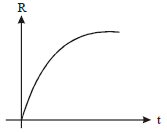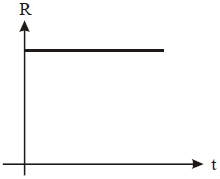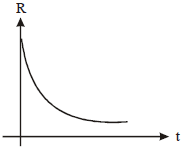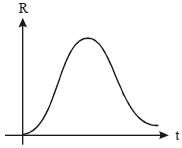The activity of the radioactivity element decreases to one-third of the original activity ${\mathrm{A}}_{0}$ in a period of 7 years. After a further lapse of 7 years, its activity will be

a.

b.

c.

d.  $\frac{{\mathrm{A}}_{0}}{9}$

Concept Questions :-

High Yielding Test Series + Question Bank - NEET 2020

Difficulty Level:

A radioactivity sample has  nuclei at a certain time. The number of active nuclei still remaining after 4 half-lives is

a.

b.

c.

d.

Concept Questions :-

High Yielding Test Series + Question Bank - NEET 2020

Difficulty Level:

a.  73

b.  74

c.  75

d.  72

Concept Questions :-

Mass-energy equivalent
High Yielding Test Series + Question Bank - NEET 2020

Difficulty Level:

a.  c + a - b

b.  c - a - b

c.  a + b + c

d.  a + b - c

Concept Questions :-

Nuclear binding energy
High Yielding Test Series + Question Bank - NEET 2020

Difficulty Level:

a.  19.6 MeV

b.  2.4 MeV

c.  8.4 MeV

d.  17.3 MeV

Concept Questions :-

Nuclear binding energy
High Yielding Test Series + Question Bank - NEET 2020

Difficulty Level:

A radioactivity nucleus X decays to a stable nucleus 'y'. Then the graph of the rate of formation of 'y' against time 't' will be

a.b.c.d.Concept Questions :-

High Yielding Test Series + Question Bank - NEET 2020

Difficulty Level:

A stationary radioactivity nucleus of mass 210 units disintegrates into an alpha particle of mass 4 units and residual nucleus of mass 206 units. If the kinetic energy of the alpha particles is E, the kinetic energy of the residual nucleus is

a.

b.

c.

d.

Concept Questions :-

High Yielding Test Series + Question Bank - NEET 2020

Difficulty Level:

The mass number of a nucleus is

a.  sometimes equal to its atomic number

b.  sometimes less than and sometimes more than its atomic number

c.  always less than its atomic number

d.  always more than its atomic number

Concept Questions :-

Mass-energy equivalent
High Yielding Test Series + Question Bank - NEET 2020

Difficulty Level:

1.

2.

3.

4.

Concept Questions :-

Nuclear binding energy
High Yielding Test Series + Question Bank - NEET 2020

Difficulty Level:

a.

b.

c.

d.

Concept Questions :-